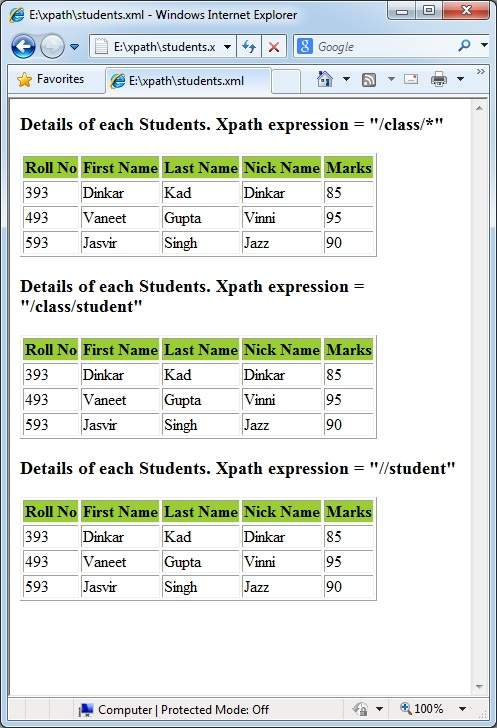# XPath - Element Node

There are multiple ways to get and handle elements.

/class/* − select all element under root node.

```<xsl:for-each select = "/class/*">
```

/class/student − select all student element under root node.

```<xsl:for-each select = "/class/student">
```

//student − select all student elements in the document.

```<xsl:for-each select = "//student">
```

## Example

In this example, we've created a sample XML document students.xml and its stylesheet document students.xsl which uses the XPath expressions.

Following is the sample XML used.

### students.xml

```<?xml version = "1.0"?>
<?xml-stylesheet type = "text/xsl" href = "students.xsl"?>
<class>
<student rollno = "393">
<firstname>Dinkar</firstname>
<nickname>Dinkar</nickname>
<marks>85</marks>
</student>
<student rollno = "493">
<firstname>Vaneet</firstname>
<lastname>Gupta</lastname>
<nickname>Vinni</nickname>
<marks>95</marks>
</student>
<student rollno = "593">
<firstname>Jasvir</firstname>
<lastname>Singh</lastname>
<nickname>Jazz</nickname>
<marks>90</marks>
</student>
</class>
```

### students.xsl

```<?xml version = "1.0" encoding = "UTF-8"?>
<xsl:stylesheet version = "1.0"
xmlns:xsl = "http://www.w3.org/1999/XSL/Transform">

<xsl:template match = "/">
<html>
<body>
<h3>Details of each Students. Xpath expression = "/class/*"</h3>

<table border = "1">
<tr bgcolor = "#9acd32">
<th>Roll No</th>
<th>First Name</th>
<th>Last Name</th>
<th>Nick Name</th>
<th>Marks</th>
</tr>

<xsl:for-each select = "/class/*">
<tr>
<td><xsl:value-of select = "@rollno"/></td>
<td><xsl:value-of select = "firstname"/></td>
<td><xsl:value-of select = "lastname"/></td>
<td><xsl:value-of select = "nickname"/></td>
<td><xsl:value-of select = "marks"/></td>
</tr>
</xsl:for-each>
</table>

<h3>Details of each Students. Xpath expression = "/class/student"</h3>

<table border = "1">
<tr bgcolor = "#9acd32">
<th>Roll No</th>
<th>First Name</th>
<th>Last Name</th>
<th>Nick Name</th>
<th>Marks</th>
</tr>

<xsl:for-each select = "/class/student">
<tr>
<td><xsl:value-of select = "@rollno"/></td>
<td><xsl:value-of select = "firstname"/></td>
<td><xsl:value-of select = "lastname"/></td>
<td><xsl:value-of select = "nickname"/></td>
<td><xsl:value-of select = "marks"/></td>
</tr>
</xsl:for-each>
</table>

<h3>Details of each Students. Xpath expression = "//student"</h3>

<table border = "1">
<tr bgcolor = "#9acd32">
<th>Roll No</th>
<th>First Name</th>
<th>Last Name</th>
<th>Nick Name</th>
<th>Marks</th>
</tr>

<xsl:for-each select = "//student">
<tr>
<td><xsl:value-of select = "@rollno"/></td>
<td><xsl:value-of select = "firstname"/></td>
<td><xsl:value-of select = "lastname"/></td>
<td><xsl:value-of select = "nickname"/></td>
<td><xsl:value-of select = "marks"/></td>
</tr>
</xsl:for-each>
</table>

</body>
</html>
</xsl:template>
</xsl:stylesheet>
```

### Verify the outputxpath_nodes.htm